# Algebra - problems - page 10

1. CircleCircle touch two parallel lines p and q; and its center lies on a line a, which is secant of lines p and q. Write the equation of circle and determine the coordinates of the center and radius. p: x-10 = 0 q: -x-19 = 0 a: 9x-4y+5 = 0
2. GardenTrapezoid garden has parallel sides 19 m and 24 m. Its area is 193.5 square meters. What is the width of the garden?
3. Barrel 3Barrel with water has a weight 118 kg. When we get off 75% of water it has a weight 35 kg. How many kg has empty barrel?
4. Wood in the forest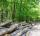The amount of wood in the forest was estimated at 6000 m3. How much wood will be in forest after 2 years if the annual growth of wood is 2.5% each year and logging 30 m3 each year?
5. Sugar - cuboid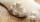Pejko received from his master cuboid composed of identical sugar cubes with count between 1000 and 2000. The Pejko eat sugar cubes in layers. The first day eat one layer from the front, second day one layer from right, the third day one layer above. Yet i
6. Coins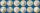Harvey had saved up a number of 2-euro coins. He stored coins in a single layer in a square. Left 6 coins. When he make square, which has one more row, missing 35 coins. How many euros he have?
7. Cylinder - hCylinder volume is 215 cm3. Base radius is 2 cm. Calculate the height of the cylinder.
8. TrioTrio of workers earn 750 euros. Money divided by the work that each of them made​​. First received twice as the second, the second received three times more than the third. How many got everyone from workers?
9. Truncated coneCalculate the height of the rotating truncated cone with volume V = 1115 cm3 and a base radii r1 = 7.9 cm and r2 = 9.7 cm.
10. Reservoir + waterReservoir completely filled with water weighs 12 kg. After pouring off three quarters of the amount of water weights 3 kg. Calculate the weight and volume of the reservoir.
11. Trains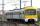On double track line between stations K and M went against each other two trains. The first train passed the distance between stations for 3.5 hours, the second, which had an average speed of 12 km/h more passed for 3.05 hours. Calculate the distance betw
12. Built-up areaJohn build up area 5 x 7 = 35 m2 with building with a wall thickness 30 cm. How many centimeters would have to subtract from thickness of the walls that built-up area fell by 9%?
13. Greek railwayman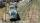Wesley works for the Slovak railways since 1986. His salary is 864 €. His colleague, Evgenias works in the Greek State Railways from 1991. Earns 5010 € per month. Calculate how many hours a day must Evgenias work to earn as much as Wesley and if they w
14. Right triangleCalculate the missing side b and interior angles, perimeter and area of ​​a right triangle if a=10 cm and hypotenuse c = 16 cm.
15. MistakeNicol mistake when calculate in school. Instead of add number 20 subtract it. What is the difference between the result and the right result?
16. GroupGroup of kids wanted to ride. When the children were divided into groups of 3 children 1 remain. When divided into groups of 4 children 1 remain. When divided into groups of 6 children 1 missed. After divided to groups of 5 children its OK. How many are t
17. Sphere in coneA sphere of radius 3 cm desribe cone with minimum volume. Determine cone dimensions.
18. Moving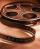Vojta left the house at three o'clockat 4 km/h. After half hour later went from the same place Filip by bicycle at speed 18 km/h. How long take Tilip to catch up Vojta and how far from the house?
19. RhombusInternal angles of rhombus is in ratio 2:3. How many times is the shorter diagonal longer than side of rhombus?
20. Camp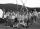In a class are 26 children. During the holidays 16 children were in the camps and 14 children on holiday with their parents. Determine the minimum and maximum number of children that may have been in the camp and on holiday with their parents at the same

Do you have an interesting mathematical problem that you can't solve it? Enter it, and we can try to solve it.

To this e-mail address, we will reply solution; solved examples are also published here. Please enter e-mail correctly and check whether you don't have a full mailbox.